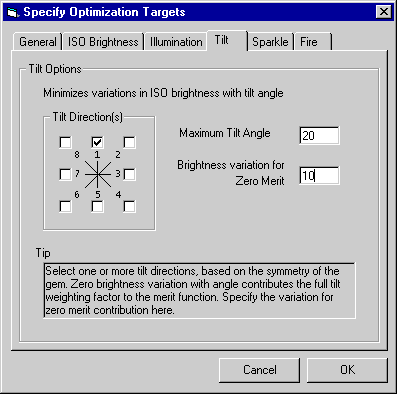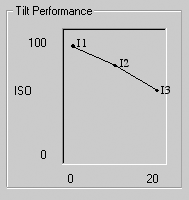### Clarification of the Tilt Performance Optimization Target

Tilt performance is among the most important criteria for evaluating the quality of a gem. Very few stones are viewed from exactly above their tables, and certainly not with uniform lighting conditions. The Tilt Performance Optimization Target forces BOG to consider the variation in brightness with tilt angle in the Merit Function.

The Tilt Performance Tutorial demonstrates the use of this Optimization Target. This document provides more information on how BOG evaluates Tilt Performance. The following clarification also applies to the Tilt Performance panel in the PlayPen.

 To quantify Tilt Performance, BOG evaluates the ISO brightness at three angles corresponding to face-up, half the specified angle, and the total specified angle. For example, if the user enters 20 degrees in the Tilt Panel of the Specify Optimization Targets window as shown at right, BOG evaluates the ISO brightness at zero, ten, and twenty degrees. At the same time, the user must specify how bad the variation must be before Tilt Performance stops contributing to the Merit Function. The Brightness Variation for Zero Merit (BVZM) sets the maximum variation. In the case shown here, the user has entered 10 percent.BOG calls GemRay three times to raytrace and evaluate the ISO brightness at each of the three angles. Let's call the corresponding ISO brightnesses I1, I2, and I3 as shown at left. The Average ISO brightness Iavg is just the sum of I1+I2+I3 divided by three: Iavg = (I1 + I2 + I3) / 3 This is the value reported in the Avg ISO box in the PlayPen. To quantify the variation in brightness with angle, BOG evaluates how far each brightness value I1,I2,I3 deviates from the average. The deviations in brightness are given by: D1 = abs(I1 - Iavg) D2 = abs(I2 - Iavg) D3 = abs(I3 - Iavg) Note that abs(x) refers to the absolute value of x, and is always a positive number. For example, abs(5-3) and abs(3-5) are both +2.

The average deviation Davg is simply the average of the individual deviations:

Davg = (D1 + D2 + D3) / 3

This is the value reported in the Var box in the PlayPen.

If Davg is zero, then I1=I2=I3, and there is no variation in brightness with tilt angle. BOG considers this the ideal situation and assigns maximum value to the Tilt Merit when this is the case. Inevitably, however, there will be some variation in brightness with angle, and hence Davg will be a positive number. If Davg exceeds the Brightness Variation for Zero Merit (BVZM), BOG assigns zero value to the Tilt Merit. If the variation lies between zero and Davg, then BOG adds an intermediate Tilt contribution to the Merit Function.

Mathematically, the Tilt Performance contribution to the overall merit function is:

Tilt Merit = TiltWeight * (1 - Davg/BVZM)

where TiltWeight is the weighting factor assigned in the General panel of the Specify Optimization Targets window.

Back to Main Page.